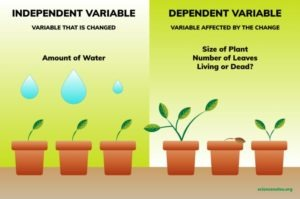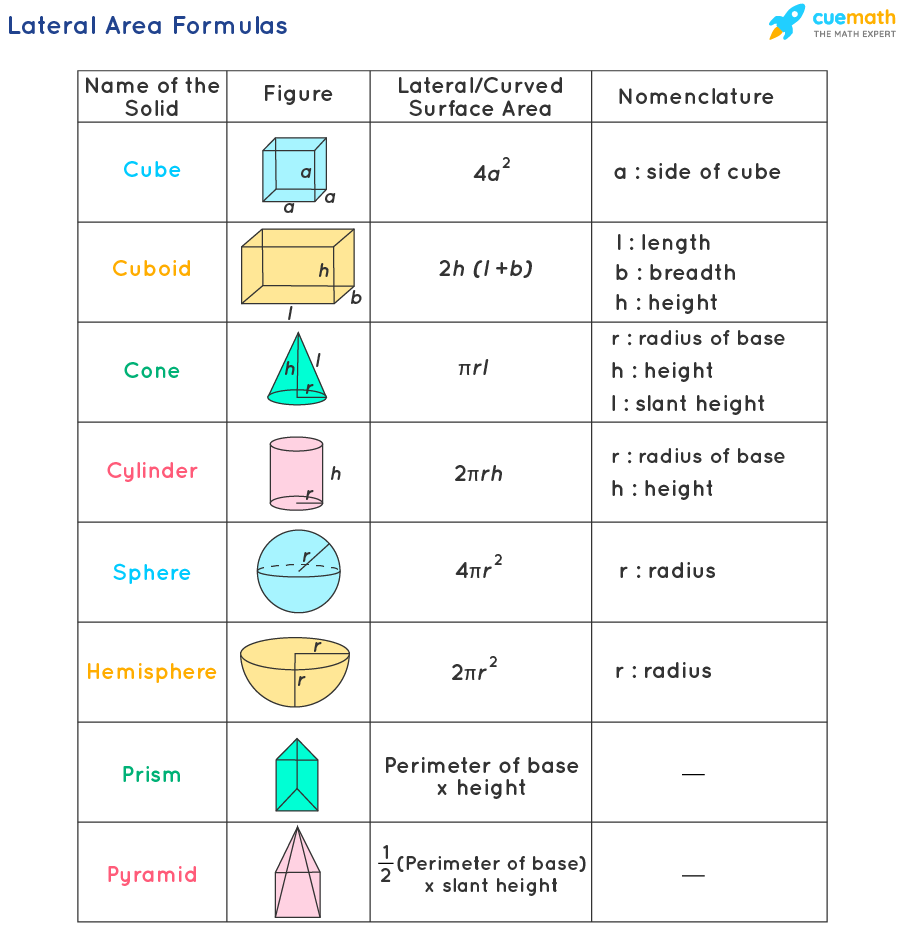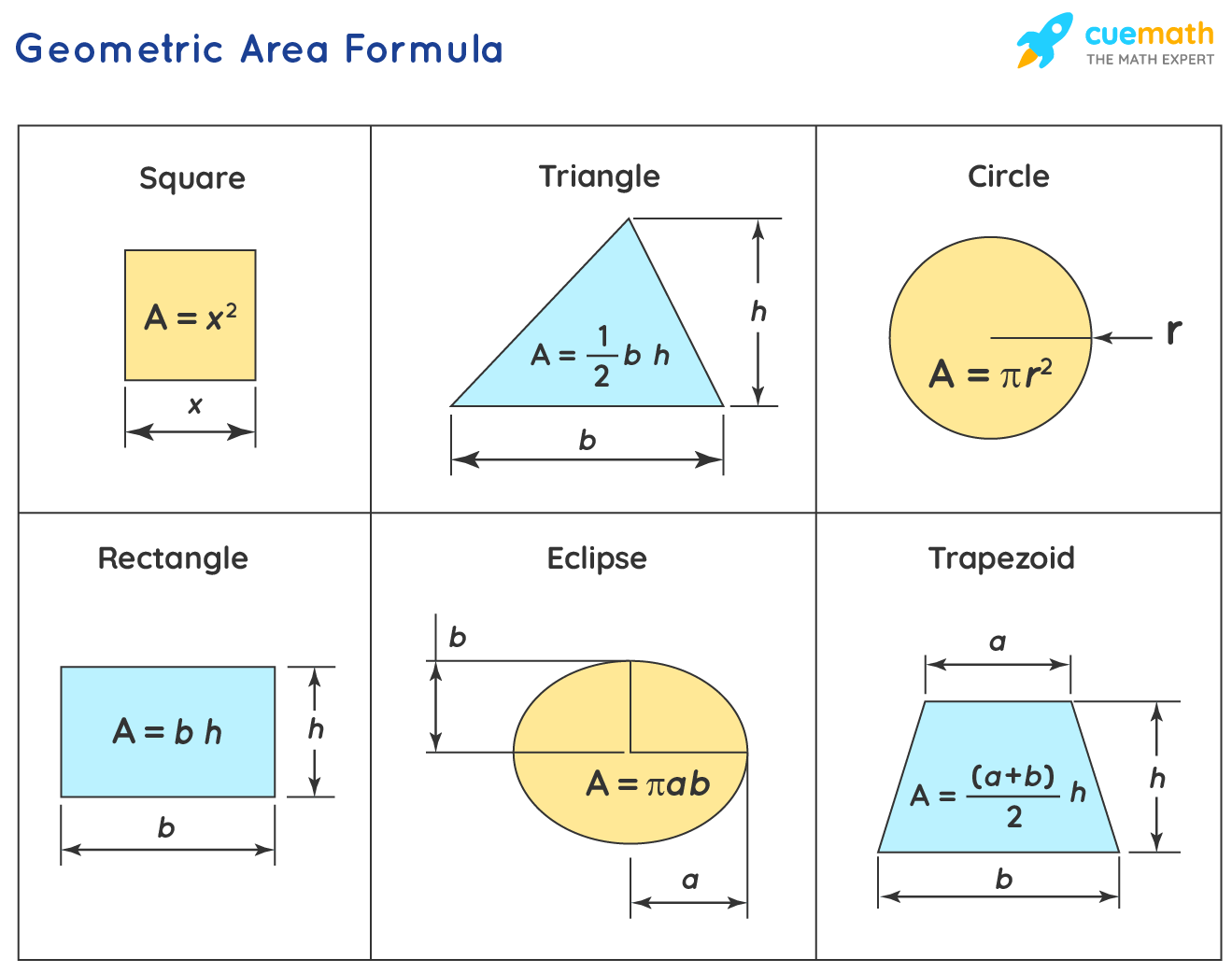## MS Math Weeks of March 21-25 & March 28-April 1Karen Evans Photography

Happy Spring!

This post covers the next two weeks until spring break.  Have a great spring break!

6th Grade Math – Mrs. Evans

This week we will finish Chapter 8 with a review and test, however we will continue our study of algebra.  In Chapter 9 we will see how two variables relate to each other in a proportional equation.  One variable is dependent on the other.  We can set up equations, tables and graphs to show proportional (or direct) relationship between two variables.  We will be investigating these skills, finishing up before spring break next week.

We can understand independent and dependent variables by viewing this spring-like picture below.  We can set up a relationship between the amount of water we give our plants and the status of the leaves:Math Grade Math – Mrs. Evans and Mrs. VonFeldt

The 7th graders will continue their study of geometry, practicing finding the surface area and volume of prisms and compound solids.  One very important skill we have been practicing in this module is using formulae.  We will finish Module 9 this week, do a review and test early next week.  We will finish up before spring break with a geometry project.  Here is a video to help with finding the volume of prisms:

8th Grade Math – Mrs. Ernest

The 8th graders will be practicing their understanding of volume and area of complex figures. Students will be building paper villages and calculating the surface area and volume of their entire village!Algebra – Mrs. VonFeldt

This week students are learning to factor trinomials (quadratic expressions). Quadratic equations are very useful in real life. Some examples include: area calculations for plots of land, calculating speed, height, or distance involving projectile motion, and genetics. We will model factoring with tiles and then look for patterns to help us develop an algorithm to factor many kinds of quadratics. The following Khan Academy video is a helpful introduction to this concept:

© 2023 Assumption Catholic School | 2116 Cornwall Ave, Bellingham, WA 98225
Phone: 360.733.6133 | Fax: 360.647.4372
Email: theoffice@school.assumption.org
Dashboard | Web design and development by Olywebdev.com